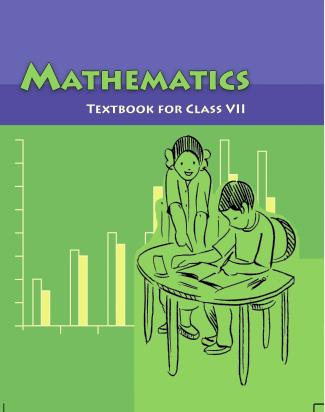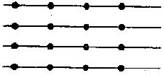1. /
2. CBSE
3. /
4. Class 07
5. /
6. Mathematics
7. /
8. NCERT Solutions for Class...

# NCERT Solutions for Class 7 Maths Exercise 2.3### myCBSEguide App

Download the app to get CBSE Sample Papers 2023-24, NCERT Solutions (Revised), Most Important Questions, Previous Year Question Bank, Mock Tests, and Detailed Notes.

NCERT solutions for Maths Fractions and Decimals## NCERT Solutions for Class 7 Maths Fractions and Decimals

###### Question 1.Find:

(i) {tex}\frac{1}{4}{/tex} of

(a) {tex}\frac{1}{4}{/tex}

(b) {tex}\frac{3}{5}{/tex}

(c) {tex}\frac{4}{3}{/tex}

(ii) {tex}\frac{1}{7}{/tex} of

(a) {tex}\frac{2}{9}{/tex}

(b) {tex}\frac{6}{5}{/tex}

(c) {tex}\frac{3}{{10}}{/tex}

(i)

(a) {tex}\frac{1}{4}{/tex} of {tex}\frac{1}{4}{/tex} = {tex}\frac{1}{4} \times \frac{1}{4} = \frac{{1 \times 1}}{{4 \times 4}} = \frac{1}{{16}}{/tex}

(b) {tex}\frac{1}{4}{/tex} of {tex}\frac{3}{5}{/tex} = {tex}\frac{1}{4} \times \frac{3}{4} = \frac{{1 \times 3}}{{4 \times 4}} = \frac{3}{{16}}{/tex}

(c) {tex}\frac{1}{4}{/tex} of {tex}\frac{4}{3}{/tex} = {tex}\frac{1}{4} \times \frac{4}{3} = \frac{{1 \times 4}}{{4 \times 3}} = \frac{1}{3}{/tex}

(ii)

(a) {tex}\frac{1}{7}{/tex} of {tex}\frac{2}{9}{/tex} = {tex}\frac{1}{7} \times \frac{2}{9} = \frac{{1 \times 2}}{{7 \times 9}} = \frac{2}{{63}}{/tex}

(b) {tex}\frac{1}{7}{/tex} of {tex}\frac{2}{9}{/tex} = {tex}\frac{1}{7} \times \frac{6}{5} = \frac{{1 \times 6}}{{7 \times 5}} = \frac{6}{{35}}{/tex}

(c) {tex}\frac{1}{7}{/tex} of {tex}\frac{2}{9}{/tex} = {tex}\frac{1}{7} \times \frac{3}{{10}} = \frac{{1 \times 3}}{{7 \times 10}} = \frac{3}{{70}}{/tex}

NCERT Solutions for Class 7 Maths Exercise 2.3

###### Question 2.Multiply and reduce to lowest form (if possible):

(i) {tex}\frac{2}{3} \times 2\frac{2}{3}{/tex}

(ii) {tex}\frac{2}{7} \times \frac{7}{9}{/tex}

(iii) {tex}\frac{3}{8} \times \frac{6}{4}{/tex}

(iv) {tex}\frac{9}{5} \times \frac{3}{5}{/tex}

(v) {tex}\frac{1}{3} \times \frac{{15}}{8}{/tex}

(vi) {tex}\frac{{11}}{2} \times \frac{3}{{10}}{/tex}

(vii) {tex}\frac{4}{5} \times \frac{{12}}{7}{/tex}

(i) {tex}\frac{2}{3} \times 2\frac{2}{3} = \frac{2}{3} \times \frac{8}{3} = \frac{{2 \times 8}}{{3 \times 3}} = \frac{{16}}{9} = 1\frac{7}{9}{/tex}

(ii) {tex}\frac{2}{7} \times \frac{7}{9} = \frac{{2 \times 7}}{{7 \times 9}} = \frac{2}{9}{/tex}

(iii) {tex}\frac{3}{8} \times \frac{6}{4} = \frac{{3 \times 6}}{{8 \times 4}} = \frac{{3 \times 3}}{{8 \times 2}} = \frac{9}{{16}}{/tex}

(iv) {tex}\frac{9}{5} \times \frac{3}{5} = \frac{{9 \times 3}}{{5 \times 5}} = \frac{{27}}{{25}} = 1\frac{2}{{25}}{/tex}

(v) {tex}\frac{1}{3} \times \frac{{15}}{8} = \frac{{1 \times 15}}{{3 \times 8}} = \frac{{1 \times 5}}{{1 \times 8}} = \frac{5}{8}{/tex}

(vi) {tex}\frac{{11}}{2} \times \frac{3}{{10}} = \frac{{11 \times 3}}{{2 \times 10}} = \frac{{33}}{{20}} = 1\frac{3}{{20}}{/tex}

(vii) {tex}\frac{4}{5} \times \frac{{12}}{7} = \frac{{4 \times 12}}{{5 \times 7}} = \frac{{48}}{{35}} = 1\frac{{13}}{{35}}{/tex}

NCERT Solutions for Class 7 Maths Exercise 2.3

###### Question 3.Multiply the following fractions:

(i) {tex}\frac{2}{5} \times 5\frac{1}{4}{/tex}

(ii) {tex}6\frac{2}{5} \times \frac{7}{9}{/tex}

(iii) {tex}\frac{3}{2} \times 5\frac{1}{3}{/tex}

(iv) {tex}\frac{5}{6} \times 2\frac{3}{7}{/tex}

(v) {tex}3\frac{2}{5} \times \frac{4}{7}{/tex}

(vi) {tex}2\frac{3}{5} \times 3{/tex}

(vii) {tex}3\frac{4}{7} \times \frac{3}{5}{/tex}

(i) {tex}\frac{2}{5} \times 5\frac{1}{4} = \frac{2}{5} \times \frac{{21}}{4} = \frac{{2 \times 21}}{{5 \times 4}} = \frac{{1 \times 21}}{{5 \times 2}} = \frac{{21}}{{10}} = 2\frac{1}{{10}}{/tex}

(ii) {tex}6\frac{2}{5} \times \frac{7}{9} = \frac{{32}}{5} \times \frac{7}{9} = \frac{{32 \times 7}}{{5 \times 9}} = \frac{{224}}{{45}} = 4\frac{{44}}{{45}}{/tex}

(iii) {tex}\frac{3}{2} \times 5\frac{1}{3} = \frac{3}{2} \times \frac{{16}}{3} = \frac{{48}}{6} = 8{/tex}

(iv) {tex}\frac{5}{6} \times 2\frac{3}{7} = \frac{5}{6} \times \frac{{17}}{7} = \frac{{85}}{{42}} = 2\frac{1}{{42}}{/tex}

(v) {tex}3\frac{2}{5} \times \frac{4}{7} = \frac{{17}}{7} \times \frac{4}{7} = \frac{{68}}{{35}} = 1\frac{{33}}{{35}}{/tex}

(vi) {tex}2\frac{3}{5} \times 3 = \frac{{13}}{5} \times \frac{3}{1} = \frac{{13 \times 3}}{{5 \times 1}} = \frac{{39}}{5} = 7\frac{4}{5}{/tex}

(vii) {tex}3\frac{4}{7} \times \frac{3}{5} = \frac{{25}}{7} \times \frac{3}{5} = \frac{{5 \times 3}}{{7 \times 1}} = \frac{{15}}{7} = 2\frac{1}{7}{/tex}

NCERT Solutions for Class 7 Maths Exercise 2.3

###### Question 4.Which is greater:

(i){tex}\frac{2}{7}{/tex} of {tex}\frac{3}{4}{/tex} or {tex}\frac{3}{5}{/tex} of {tex}\frac{5}{8}{/tex}

(ii){tex}\frac{1}{2}{/tex} of {tex}\frac{6}{7}{/tex} or {tex}\frac{2}{3}{/tex} of {tex}\frac{3}{7}{/tex}

(i) {tex}\frac{2}{7}{/tex} of {tex}\frac{3}{4}{/tex} or {tex}\frac{3}{5}{/tex} of {tex}\frac{5}{8}{/tex} {tex} \Rightarrow {/tex} {tex}\frac{2}{7}{/tex} x {tex}\frac{3}{4}{/tex} or {tex}\frac{3}{5}{/tex} x {tex}\frac{5}{8}{/tex}

{tex} \Rightarrow {/tex} {tex}\frac{3}{{14}}{/tex} or {tex}\frac{3}{8}{/tex} {tex} \Rightarrow {/tex} {tex}\frac{3}{{14}} < \frac{3}{8}{/tex}

Thus, {tex}\frac{3}{5}{/tex} of {tex}\frac{5}{8}{/tex} is greater.

(ii) {tex}\frac{1}{2}{/tex} of {tex}\frac{6}{7}{/tex} or {tex}\frac{2}{3}{/tex} of {tex}\frac{3}{7}{/tex} {tex} \Rightarrow {/tex} {tex}\frac{1}{2}{/tex} x {tex}\frac{6}{7}{/tex} or {tex}\frac{2}{3}{/tex} x {tex}\frac{3}{7}{/tex}

{tex} \Rightarrow {/tex} {tex}\frac{3}{7}{/tex} or {tex}\frac{2}{7}{/tex} {tex} \Rightarrow {/tex} {tex}\frac{3}{7}{/tex}>{tex}\frac{2}{7}{/tex}

Thus, {tex}\frac{1}{2}{/tex} of {tex}\frac{6}{7}{/tex} is greater.

NCERT Solutions for Class 7 Maths Exercise 2.3

###### Question 5.Saili plants 4 saplings in a row in her garden. The distance between two adjacent saplings is {tex}\frac{3}{4}{/tex} m. Find the distance between the first and the last sapling.

The distance between two adjacent saplings = {tex}\frac{3}{4}{/tex} m

Saili planted 4 saplings in a row, then number of gap in saplingsTherefore, the distance between the first and the last saplings = {tex}3 \times \frac{3}{4}{/tex} = {tex}\frac{9}{4}{/tex} m = {tex}2\frac{1}{4}{/tex} m

Thus the distance between the first and the last saplings is {tex}2\frac{1}{4}{/tex} m.

NCERT Solutions for Class 7 Maths Exercise 2.3

###### Question 6.Lipika reads a book for {tex}1\frac{3}{4}{/tex} hours everyday. She reads the entire book in 6 days. How many hours in all were required by her to read the book?

Time taken by Lipika to read a book = {tex}1\frac{3}{4}{/tex} hours.

She reads entire book in 6 days.

Now, total hours taken by her to read the entire book = {tex}1\frac{3}{4} \times 6{/tex} = {tex}\frac{7}{4} \times 6 = \frac{{21}}{2} = 10\frac{1}{2}{/tex} hours

Thus {tex}10{/tex} hours were required by her to read the book.

NCERT Solutions for Class 7 Maths Exercise 2.3

###### Question 7.A car runs 16 km using 1 litre of petrol. How much distance will it cover using {tex}2\frac{3}{4}{/tex} litres of petrol?

In 1 litre of pertrol, car covers the distance = 16 km

In {tex}2\frac{3}{4}{/tex} litres of petrol, car covers the distance = {tex}2\frac{3}{4}{/tex} of 16 km = {tex}\frac{{11}}{4} \times 16{/tex} = 44 km

Thus, car will cover 44 km distance.

NCERT Solutions for Class 7 Maths Exercise 2.3

###### Question 8.(a) (i) Provide the number in the box {tex}\boxed{{\text{ }}},{/tex} such that {tex}\frac{2}{3} \times \boxed{{\text{ }}} = \frac{{10}}{{30}}.{/tex}

(ii) The simplest form of the number obtained in {tex}\boxed{{\text{ }}}{/tex} is __________.

(b) (i) Provide the number in the box {tex}\boxed{{\text{ }}},{/tex} such that {tex}\frac{3}{5} \times \boxed{{\text{ }}} = \frac{{24}}{{75}}.{/tex}

(ii) The simplest form of the number obtained in {tex}\boxed{{\text{ }}}{/tex} is __________.

(a)

(i) {tex}\frac{2}{3} \times \boxed{\frac{5}{{10}}} = \frac{{10}}{{30}}{/tex}

(ii) The simplest form of {tex}\frac{5}{{10}}{/tex} is {tex}\frac{1}{2}.{/tex}

(b)

(i) {tex}\frac{3}{5} \times \boxed{\frac{8}{{15}}} = \frac{{24}}{{75}}{/tex}

(ii) The simplest form of {tex}\frac{8}{{15}}{/tex} is {tex}\frac{8}{{15}}.{/tex}

## NCERT Solutions for Class 7 Maths Exercise 2.3

NCERT Solutions Class 7 Maths PDF (Download) Free from myCBSEguide app and myCBSEguide website. Ncert solution class 7 Maths includes text book solutions from Class 7 Maths Book . NCERT Solutions for CBSE Class 7 Maths have total 15 chapters. 7 Maths NCERT Solutions in PDF for free Download on our website. Ncert Maths class 7 solutions PDF and Maths ncert class 7 PDF solutions with latest modifications and as per the latest CBSE syllabus are only available in myCBSEguide.

## CBSE app for Students

To download NCERT Solutions for Class 7 Maths, Social Science Computer Science, Home Science, Hindi English, Maths Science do check myCBSEguide app or website. myCBSEguide provides sample papers with solution, test papers for chapter-wise practice, NCERT solutions, NCERT Exemplar solutions, quick revision notes for ready reference, CBSE guess papers and CBSE important question papers. Sample Paper all are made available through the best app for CBSE students and myCBSEguide website.Test Generator

Create question paper PDF and online tests with your own name & logo in minutes.myCBSEguide

Question Bank, Mock Tests, Exam Papers, NCERT Solutions, Sample Papers, Notes

### 2 thoughts on “NCERT Solutions for Class 7 Maths Exercise 2.3”

1. I like this website

2. This is a very good website i like it you should definetly try thos website